Computational & Technology Resources an online resource for computational,engineering & technology publications not logged in - login Civil-Comp ProceedingsISSN 1759-3433 CCP: 84PROCEEDINGS OF THE FIFTH INTERNATIONAL CONFERENCE ON ENGINEERING COMPUTATIONAL TECHNOLOGY Edited by: B.H.V. Topping, G. Montero and R. Montenegro Paper 32Modelling of Forest Fire Spreading by Using Hexagonal Cellular Automata A. Hernández Encinas1, L. Hernández Encinas2, S. Hoya White1, A. Martín del Rey1 and G. Rodríguez Sánchez11Department of Applied Mathematics, University of Salamanca, Spain 2Department of Information Processing and Coding, C.S.I.C., Spain doi:10.4203/ccp.84.32 Full Bibliographic Reference for this paper A. Hernández Encinas, L. Hernández Encinas, S. Hoya White, A. Martín del Rey, G. Rodríguez Sánchez, "Modelling of Forest Fire Spreading by Using Hexagonal Cellular Automata", in B.H.V. Topping, G. Montero, R. Montenegro, (Editors), "Proceedings of the Fifth International Conference on Engineering Computational Technology", Civil-Comp Press, Stirlingshire, UK, Paper 32, 2006. doi:10.4203/ccp.84.32 Keywords: fire spreading, cellular automata, hexagonal lattice, mathematical modelling. Summary As is well-known, forest fires are one of the major ecological agents in forest since they alter the structure and composition of forest and adversely affect human health and the supply of goods and services on which communities depend. Consequently, the study of forest fires have received increased public attention by the scientific community. Specifically, several mathematical models have appeared in the literature in order to study the spreading of forest fire fronts. The efforts to model the growth of the fire front by means of mathematical models can be classified according to two approaches: vector models and cellular automata models. Vector models assume that the fire front spreads according to a well-defined growth law, and take a standard geometrical shape (see, for example, Vakalis et al. ). On the other hand, several types of cellular automata models for fire spreading have been introduced in the literature (see Karafyllidis et al. [1,2]). The main goal of this work is to introduce a new discrete mathematical model for predicting the behaviour of the forest fire front based on a two-dimensional hexagonal cellular automata. Basically, it consists of a two-dimensional hexagonal array of a finite number of identical objects called cells, interacting locally with each other. Each cell can assume a state from a finite or infinite state set, which changes at every time step according to a fixed rule. The variables of such function are the states of some neighbour cells at the previous time step. The proposed model divides the forest into regular hexagonal areas, each one of them stands for a cell. The state of each cell is a real number from the interval [0,1]. If it is 0, then the cell is unburned, and if the state is 1, the cell is totally burned out. The evolution rule linearly depends on thirteen variables: the states of the twelve nearest cells to a given one and its own state. These variables are affected by some parameters which stand for wind speed and the topography. A forest area can be interpreted as the hexagonal cellular space of a CA by simply dividing it into a bidimensional array of identical regular hexagonal areas of side length L. Obviously, each one of these areas stands for a cell of the CA. The state of a cell (a,b) at a time t, is defined as follows: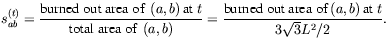If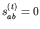, then the cell (a,b) is said to be unburned at time t; if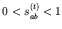, the cell (a,b) is partially burned out at time t, and finally, if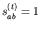, the cell is said to be completely burned out at time t. The evolution of the CA used in the proposed model supposes that the state of the cell (a,b) at time t+1 linearly depends on the states of its neighbor cells at time t. In the proposed model, we will consider a finite state set of order 101k, that is,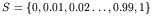. Then, a suitable discretization function,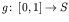, must be incorporated to the model as follows: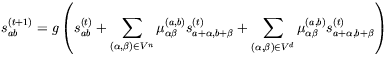where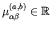are parameters involving some physical magnitudes of the cells: the topography, the weather conditions, the rate speed associated to the combustible of the cell, etc. The simulations obtained seem to be in agreement with real simulations given by real data. References 1 I. Karafyllidis, "Design of a dedicated parallel processor for the prediction of forest fire spreading using cellular automata and genetic algorithms", Engineering Applications of Artificial Intelligence, 17, 19-36, 2004. doi:10.1016/j.engappai.2003.12.001 2 I. Karafyllidis, A. Thanailakis, "A model for predicting forest fire spreading using cellular automata", Ecological Modelling, 99, 87-97, 1997. doi:10.1016/S0304-3800(96)01942-4 3 D. Vakalis, H. Sarimveis, C. Kiranoudis, C., A. Alexandridis, G. Bafas, "A GIS based operational system for wildland fire crisis management I. Mathematical modelling and simulation", Applied Mathematical Modellling, 28, 389-410, 2004. doi:10.1016/j.apm.2003.10.005 purchase the full-text of this paper (price £20) Back to top ©Civil-Comp Limited 2020 - terms & conditions